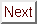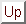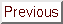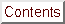Next: Possible Interpretations of Up: Upper Critical Field Previous: Microscopic Models of

# Experimental Results: Temperature Dependence of the Upper Critical Field

Many of the experimental factors relevant to the angular behavior of the critical field will also complicate the temperature dependence. Among these are sample misalignment, mosaic spread, and critical field definition. In addition there are the considerations of temperature measurement, stability, and equilibration. (See Section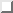.) Nonetheless, the Hc2(T) results are a bit easier to interpret than the Hc2(theta) ones, if only because the functional form of the data is simpler. The approximately linear behavior of the temperature dependence curves is illustrated in Figure, which shows Hc2(T) curves for four different C4KHg GIC's. These data are from the same samples whose Hc2(theta) curves are shown in Figure.Figure: Critical field Hc2 as a function of reduced temperature for C4KHg. Dotted curves are least-squares line fits to the data. Fit parameters are given in Table. a) Data for a C4KHg with Tc = 0.95 K: (circ), vecH _|_ ^c. (bullet), vecH || ^c Data for a Tc = 0.73 K sample from Ref. : (diamond), vecH _|_ ^c. (×), vecH || ^c. b) Data for two C4KHg-GIC's with Tc 1.5 K. Tc = 1.53 K sample: (circ), vecH _|_ ^c. (bullet), vecH || ^c. Tc = 1.54 K sample: diamond, vecH _|_ ^c. ×, vecH || ^c.

The fits in Figureare all to the simple linear formwhere t is the reduced temperature, T/ Tc. The least-squares-determined values of Hc2(0) and Tc for these curves are given in Table. The quality-of-fit parameter cal R is calculated as in Eqn., only here the error at the ith point sigmai is taken to be the same as the experimental field value, that is:Of course the errors should really be smaller in magnitude than the data, but their fractional size is thought to be roughly independent of temperature, the independent variable. Letting the estimated error be the same as the data is a convenient way of implementing this standard assumption, which is called statistical weighting. The field error bars shown on the plot are twice the mean error per data point in total length, where the mean error sigma is given bywhere nu is the number of degrees of freedom. This is a standard way of defining error bars which includes the effects only of random errors, not systematic ones. The temperature error bars are based on an uncertainty of 40 mK at the low end, and 10 mK at the high end, for reasons discussed in Section.Table: Parameters obtained from least-squares fits of Eqn.to C4KHg Hc2(T) data. The individual specimens are labeled by the value of Tc obtained from the zero-field sweeps. The other parameters, including the ``fit'' Tc, are obtained from the least-squares linear fits to the critical field data that are shown in Figure. The residual cal R is calculated using Eqn.. NR denotes that the parameter was not reported in the cited reference.

In general, the data in Figureare quite well described by Eqn.. Ginzburg-Landau models predict a linear temperature-dependence of Hc2 in their region of validity, so this result is not surprising, especially since Iye and Tanuma found linear temperature dependence for their C4KHg samples. One feature of Tablethat merits some discussion is that the Tc determined from the best linear fit is as much as 10% higher than that determined from the zero-field temperature sweeps. This disparity between the different ways of measuring Tc is a fairly common occurrence in superconductivity which is generally caused by curvature of the Hc2(T) data in the region near Tc. Hohenberg and Werthamer found that Fermi-surface anisotropy can cause positive curvature of the critical field near Tc, but here negative curvature is needed in order to explain the high extrapolated values of Tc.

For the higher- Tc specimens, the Tc numbers found from the vecH || ^c fits are higher than those found from the vecH _|_ ^c fits. Because of the discussion of type I superconductivity in the Section, one might wonder if the Hc2, || ^c(T) data in these samples are actually quadratic, since a quadratic temperature dependence is expected for measurements of the thermodynamic critical field. A moment's thought indicates that a linear fit to a quadratic function will have a higher intercept on the x-axis than a quadratic fit will. (Se Figure.) Therefore, if the data are truly quadratic, it is easy to see why a linear fit will give a falsely high Tc. The temperature dependence data for the Tc 1.5 K GIC's for vecH ||^c are shown on an expanded scale in Figure, along with the best quadratic and linear fits. This plot is quite similar to Figure, where the presumed thermodynamic critical field values were obtained from fits to Hc2(theta). Here the data were obtained directly, using field sweeps at constant theta as a function of temperature.Figure: Critical fields with vecH || ^c for Tc 1.5 K C4KHg samples. Uparrow marks the value of Tc found using a zero-field temperature sweep. a) (bullet), data for a Tc = 1.53 K sample; (diamond), a linear fit to the data with Hc2(0) = 89.7 Oe, Tc = 1.65 K and cal R = 6.25e-3; (.), a quadratic fit to the data with Hc2(0) = 64.0 Oe, Tc = 1.55 K and cal R = 1.2e-2. b) (bullet), data for a Tc = 1.54 K sample; (diamond), a linear fit to the data with Hc2(0) = 85.8 Oe, Tc = 1.62 K and cal R = 1.62e-3; (.), a quadratic fit to the data with Hc2(0) = 62.8 Oe, Tc = 1.51 K and cal R = 4.7e-2.

Figureshows that using a quadratic function to fit the vecH || ^c data does improve the agreement between the fit Tc and that obtained from a zero-field sweep. Unfortunately, the quadratic function does not describe the data as well as a linear function: for one sample the residual for the quadratic fit is twice that of the linear fit, and for the other it is three times that of the linear fit. Consultation of standard tables shows that the difference between these fits has only about an even chance of being significant (for about 15 data points). Therefore the only safe statement is that the question of type I superconductivity in C4KHg is still unresolved, since the fits to Hc2, || ^c are inconclusive. As was mentioned in relation to Figure, in C8K both Hc(T) and Hc2,_|_ ^c(T) show positive curvature, so perhaps in C4KHg it is not implausible for both critical fields to show a linear temperature dependence.

Some lower- Tc samples, such as the Tc = 0.95 K specimen whose data is shown in Figure(and others whose data are not displayed) showed Tc's which were higher than the zero-field values for both field orientations. This fact suggests that some experimental error is causing the discrepancies. Because the vecH || ^c measurements were usually taken going down in temperature, and those for vecH _|_ ^c were generally taken going up in temperature (see Section), it appears unlikely that systematic temperature measurement problems are responsible. At this time the high values of Tc obtained from the Hc2, || ^c fits remain unexplained, but it is felt that some type of measurement problem is the most likely cause.

Another unexpected feature of the data for both orientations is that the linearity of Hc2(t) persists to unusually low reduced temperatures. For typical type II superconductors, Eqn.holds only for about 0.6 < t < 1.0, below which a saturation in Hc2(t) becomes noticeable. This saturation is described quantitatively by the widely accepted theory of Werthamer, Helfand, and Hohenberg (WHH) which has been successful in describing a wide variety of superconducting materials[186,84]. Anisotropic materials which are described by the AGL model for their angular dependence are expected to have a temperature dependence at constant angle describable by the WHH theory. The basic equation of this theory is one developed by Maki and deGennes[210,108]:where t is the reduced temperature, D is the diffusivity, and the digamma function psi(x) is related to the gamma function by:This equation is strictly applicable only to dirty superconductors or clean superconductors near Tc, but the theory has been extended to lower temperatures for clean superconductors by Helfand and Werthamer. The contribution of WHH was to further extend the theory to incorporate effects due to Pauli paramagnetism and spin-orbit coupling. The meaning and possible importance of these embellishments is discussed below in Section. Simple two-parameter ( Tc, <=ft. dHc2/dT |Tc) WHH fits to the C4KHg data are shown in Figure, where they are compared to the best linear fits, the same linear fits shown in Figure.Figure: Comparison of WHH and linear fits to Hc2(T) data taken on a Tc = 1.54 K sample. a) (bullet), data for vecH _|_ ^c. (.), linear fit with Hc2(0) = 748 Oe, Tc = 1.52 K, and cal R = 6.9e-3. (circ), WHH fit with Hc2(0) = 518 Oe, Tc = 1.53 K, and cal R = 1.6e-2. b) (bullet), data with vecH || ^c. (.), linear fit with Hc2(0) = 85.8 Oe, Tc = 1.62 K, and cal R = 1.6e-3. (circ), WHH fit with Hc2(0) = 59.76 Oe, Tc = 1.63 K, and cal R = 1.2e-2.

As might be expected, the Maki-deGennes equation gives approximately linear behavior near Tc. Therefore the linear and WHH fits are in good agreement just below Tc. However, the low-temperature extension of the Maki-deGennes formalism produces the resultThis formula is the quantitative expression of the saturation shown in the WHH curves of Figure, saturation which the data does not appear to exhibit. The better agreement of the linear fits than the WHH fits is confirmed by the residual indices, which are significantly lower for the linear fits.

A more impressive demonstration of linearity is shown in Figure, which gathers together the data of 5 samples for both orientations. Here the data are plotted in dimensionless units on both axes; the reduced field h* is defined byFor these 143 data points, the residual index for the linear fit is 3/4 that of the WHH fit, indicative of about a 90 % probability that the line describes the data better. Figurealso shows that at the lowest reduced temperature the data have already reached h* 0.7, the zero-temperature value of h* calculated using the Helfand-Werthamer formalism. Therefore, while it would be gratifying to measure Hc2(t) to lower reduced temperatures and see even larger deviations from the WHH theory, the available data (down to t = 0.3) demonstrate convincingly that the observed deviations are real.Figure: Summary of all Hc2 data, both _|_ and || to the c-axis. The dimensionless quantities plotted are reduced field ( h*) versus reduced temperature (t). (bullet), 143 data points taken on 5 different GIC's. (circ), best 2-parameter WHH fit to the data with cal R = 1.7e-2. (.), best linear fit to the data with cal R = 1.3e-2. Both fits have fracdh*dt = -1 at t = 1.

The conclusions drawn here are perfectly consistent with those stated by Iye and Tanuma in their papers on C4KHg. The reason is that their data extended over a smaller temperature range than the MIT data. At the lowest reduced temperature for which they reported measurements on C4KHg, t = 0.55, linear character is consistent with both the WHH and linear fits, as Figureshows. Therefore extended linearity was unobservable in Iye and Tanuma's samples in the temperature interval in which they performed measurements. It should be noted, though, that unusual Hc2(T) behavior may not occur in the lower- Tc samples. Considering that the Hc2(theta) for the lower- Tc GIC's were well-described by the simple AGL model, it seems reasonable that the lower- Tc Hc2(T) curves may agree well with the Maki-deGennes equation.

Examination of Equationshows that there is a lot more information that can be extracted from the Hc2(T) data. To begin with, the value of epsilon, the anisotropy parameter, can be calculated asand compared with the epsilon obtained from fits to the Hc2(theta) data. The resultant numbers are displayed in Table.Table: Comparison of the anisotropy parameter epsilon as obtained from Hc2(T) and Hc2(theta) fits. The Hc2(T) epsilon numbers were obtained from the ratio of the slopes. The Hc2(theta) numbers were obtained from fits using Eqns.(AGL) and(Tinkham's formula), with type I superconductivity allowed for small theta. In each case, the TF fits had lower residuals than the AGL fits (see Table). NA indicates that a TF fit was not performed on this data; NR denotes information that was not reported in the cited reference.

As the table shows, agreement between the two methods of determining epsilon is rather poor. While epsilon as determined from Hc2(T) is consistently about 9, that determined from Hc2(theta) ranges from 8 to 16. The reason that the angular dependence's epsilon's are higher is that in fitting Hc2(theta) it has been assumed that the number measured for Hc2(0°) is actually Hc, and that the real value of Hc2(0°) is much lower. As discussed in Section, the Hc2(theta) data cannot be fit without making use of this assumption. In addition, the presence of type I superconductivity is supported by the Cv measurements done by Alexander et al.

It is tempting to conclude that the values for epsilon determined from the angular dependence are unreliable because of problems with the fits. The matter is not that simple though, since the variability of epsilon can be demonstrated in a model-independent way using the Hc2(theta) data, without benefit of fits. One way of doing this is to plot Hc2(theta)/Hc2(0°) versus theta. This scaling forces the curves through the common point (0°, 1), with the value at the peak being 1/epsilon at the temperature of measurement. If epsilon is in fact constant with respect to temperature, then the Hc2(theta)/Hc2(0°) versus theta curves should all overlay one another except for random errors.Figure: Demonstration of the temperature dependence of the anisotropy parameter epsilon in C4KHg, where 1/epsilon == Hc2(90°)/Hc2(0°). Data are for a Tc = 1.54 K C4KHg sample. (circ), t = 0.29. (bullet), t = 0.55. (×), t = 0.76. All Hc2(0°) values were determined from the data, not the fits, so that this plot is model-independent. Fits to this data are shown in Figure.

That this is not the case is demonstrated in Figure. The t = 0.55 and t = 0.76 curves appear to overlay for the most part, but the t = 0.29 curve clearly rises above the other two at the peak. Figuretherefore suggests that the variation in epsilon between t = 0.29 and t = 0.55 is real, but that any variation between t = 0.55 and t = 0.76 is at best small. From Table, one can see that the magnitudes of epsilon from the best fits (to Tinkham's formula) support this conclusion, although the verdict of the higher-residual AGL fits is less clear.

A temperature-dependent epsilon implies a temperature-dependent slope in at least one of the high-symmetry directions. To be more precise, an increase in the anisotropy at low reduced temperatures requires either a downward deviation from linearity in Hc2, || ^c or an upward deviation from linearity in Hc2, _|_ ^c. While it is certainly true that neither of these trends is obvious in the Hc2(T) data, it also turns out that neither of these is ruled out. The reason is that if the idea of type I superconductivity in C4KHg is taken seriously, then the data plotted in Figureb) are Hc rather than Hc2, and so make no statement about any hypothetical curvature of Hc2, || ^c(T). Any positive curvature of Hc2, _|_ ^c would be lost in the noise of the data at low reduced temperatures, so that any kink of the size predicted by the Hc2(theta) would be unobservable.

So what are the best numbers for epsilon(T) from the sum total of these data sets? One important point to notice is that the magnitude of the anisotropy derived from the linear fits to the Hc2(T) curves represents a sort of thermal average. Thus epsilon = 9 is probably the mean value over the range 0.3 < t < 0.95. The evidence from the angular dependence, which measures the anisotropy at a specific t, indicates that the true epsilon is higher at low reduced temperatures and lower at higher reduced temperatures. Because the Hc2(theta) data is much less affected by experimental errors, the magnitudes of epsilon determined from the Hc2(theta) fits are thought to be more reliable.

Temperature-dependent anisotropy has been observed before in graphite intercalation compounds, specifically in C8KHg by Iye and Tanuma. The increase in anisotropy from 17.6 at t = 0.81 to 21.6 at t = 0.23 is illustrated in Figure. The variation of epsilon shown there is similar to what is reported here for C4KHg. C8KHg is the only GIC for which Iye and Tanuma showed Hc2(theta) curves at more than one temperature. This is unfortunate, since it would be interesting to know whether a variable epsilon is a general property of GIC superconductors.Figure: Temperature-dependent anisotropy in C8KHg is demonstrated by a plot of Hc2(theta)/Hc2(0°) versus theta, just as in Figure. All data from Iye and Tanuma, Ref.  on a Tc = 1.94 K sample. (×), data at t = 0.23. Fit, (diamond), with 1/epsilon = 17.6 and cal R = 6.8e-3. (bullet), data at t = 0.81. (circ), fit with 1/epsilon = 21.6 and cal R = 5.3e-3.Figure: Positive curvature of Hc2(T) in C8RbHg. Data are taken from Iye and Tanuma, Ref. . (circ), Hc2, _|_ ^c. (bullet), Hc2, || ^c. Parameters for the line fits: for vecH _|_ ^c, Hc2(0) = 3078 Oe, Tc = 1.36 K, and cal R = 0.56; for vecH || ^c, Hc2(0) = 89.0 Oe, Tc = 1.37 K, and cal R = 3.02e-2. Zero-field Tc for this sample was 1.4 K.

There is some justification for speculating that a temperature-dependent anisotropy is a common property of the class. According to data shown in Ref. , all of the superconducting GIC's (with the possible exception of C4KHg) show positive curvature of their upper critical fields with respect to temperature (d Hc22/dT2 > 0). The largest curvature seems to occur in C8RbHg, as the data in Figureshow. Positive curvature does not guarantee a variable epsilon, of course, but the chances of exactly the same curvature in both critical fields seems small. In terms of the theories described in Section, identical curvature in both of the orientations would be a coincidence rather than a natural consequence of the models (although identical curvature for both orientations has been reported for at least one compound, Fe0.05TaS2). These models are discussed in more detail in the section that follows.

In the section that follows, reference will frequently be made to theories which predict positive curvature of Hc2(T). These references should not be understood as suggestions that positive curvature is observed in C4KHg, but rather as expressions of the philosophy that whatever phenomena are the cause of the positive curvature in C8RbHg are most likely also to cause the extended linearity seen in C4KHg. Theories which can produce upward-curving critical field curves should easily be able to produce straight ones through adjustment of parameters. In these models, after all, a straight Hc2(T) means merely the compensation of the forces which drive upward and downward curvature.

Before moving on to the interpretation of these results, let's pause to summarize. In C4KHg, the critical field both perpendicular and parallel to the graphite c-axis shows enhanced linearity with respect to the usual theory for type II superconductors. The data parallel to ^c are only marginally consistent with the quadratic behavior expected for the thermodynamic critical field of type I superconductors. However, Hc(T) in C8K is not well-fit by a quadratic, either. Clem has shown that superconducting energy gap anisotropy can cause a slight deviation from the BCS temperature dependence of Hc. However, the deviation expected in Clem's theory is so small that his model should not be considered a serious candidate to explain the deviations seen in GIC's. The introduction of the possibility of type I superconductivity was suggested by the specific heat data of Alexander et al., and also by the poor quality of the Hc2(theta) fits without it. The Hc2(theta) fits also suggest that the anisotropy parameter epsilon is temperature-dependent. Both the temperature dependence of epsilon and the enhanced linearity of Hc2(T) are inexplicable within the simple anisotropic Ginzburg-Landau theory,[244,155] although basically the data are well-described by this model. The AGL and other, more detailed, models are discussed at length in the next section.Next: Possible Interpretations of Up: Upper Critical Field Previous: Microscopic Models of

alchaiken@gmail.com (Alison Chaiken)
Wed Oct 11 22:59:57 PDT 1995